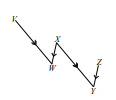PSA2PWW - Problem Sets---Geometry, M1 Lesson 33 (G.CO.1 G.CO.2 G.CO.3)
Part A)

Use any of the assumptions, facts, and/or properties presented in the tables from this lesson's review exercises to find x and y in each figure below. Justify your solutions.

Do not include units (degrees) in your solutions.

x = ___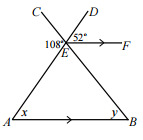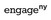Modified from EngageNY ©Great Minds Disclaimer

Type your answer below as a number (example: 5, 3.1, 4 1/2, or 3/2):
Part B)

y = ___

Type your answer below as a number (example: 5, 3.1, 4 1/2, or 3/2):
Part C)

Part A)

You need to draw an auxiliary line to solve this problem.

x = ___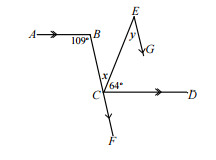Modified from EngageNY ©Great Minds Disclaimer

Type your answer below as a number (example: 5, 3.1, 4 1/2, or 3/2):
Part B)

y = ___

Type your answer below as a number (example: 5, 3.1, 4 1/2, or 3/2):
Part C)

Part A)

x = ___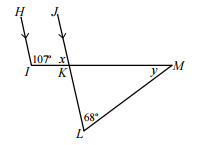Modified from EngageNY ©Great Minds Disclaimer

Type your answer below as a number (example: 5, 3.1, 4 1/2, or 3/2):
Part B)

y = ___

Type your answer below as a number (example: 5, 3.1, 4 1/2, or 3/2):
Part C)